Wednesday 17th August 2022
 All India Engineering / Architecture Entrance Examination (AIEEE) CBSE Guess > AIEEE > AIEEE Papers > 2009 > Physics AIEEE 2009 Physics Q.1. Three sound waves of equal amplitudes have frequencies (v – 1), v, (v + 1). They superpose to give beats. The number of beats produced per second will be:  1 4 3 2 Sol: Variation of intensity due to superposition of waves having frequencies v -1 & v and v & v + 1 is as shown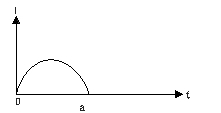Variation of intensity due to superposition of waves having frequencies v – 1 and v + 1 is as shown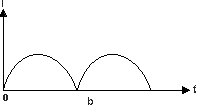Resultant of (a) and (b) be as shown So number of beats/s = 2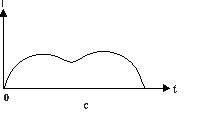Answer: (4)  Q.2. The height at which the acceleration due to gravity becomes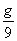(where g = the acceleration due to gravity on the surface of the earth) in terms of R, the radius of the earth is: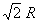2R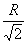R/2 Sol: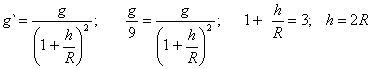Answer: (2)